Anúncio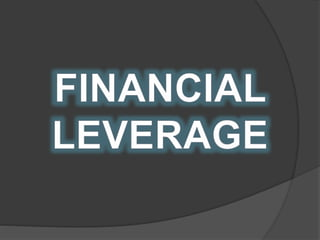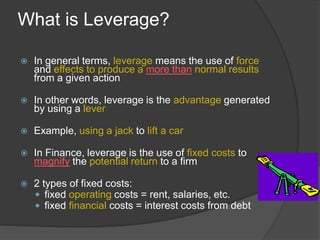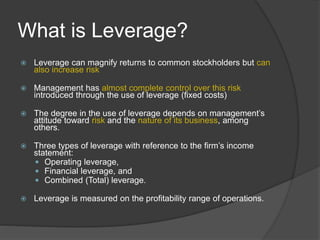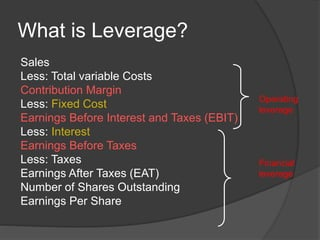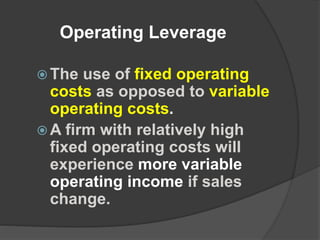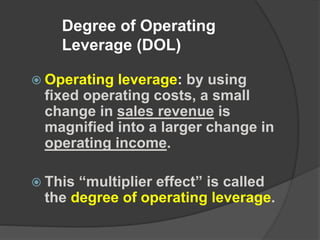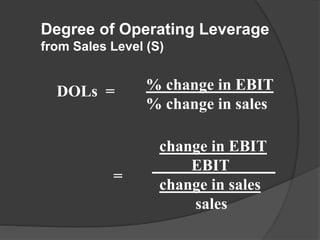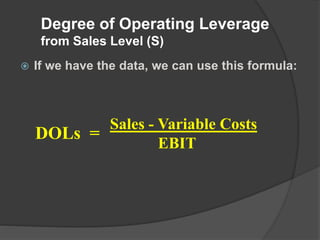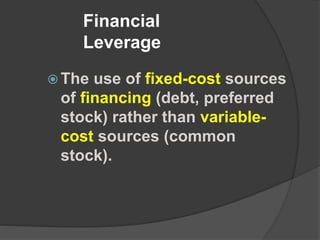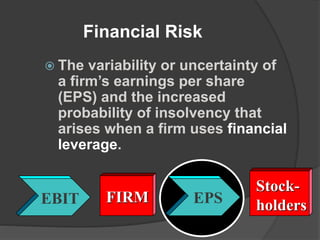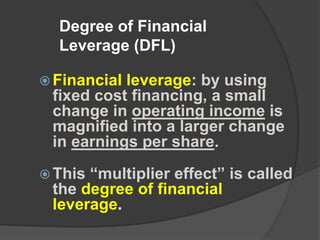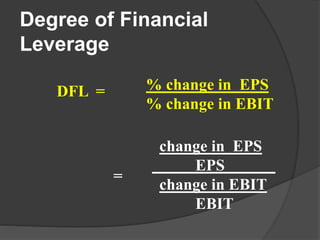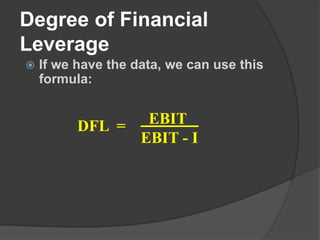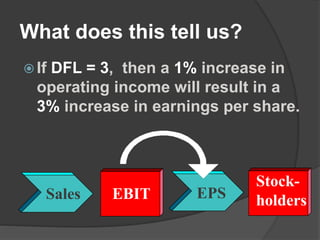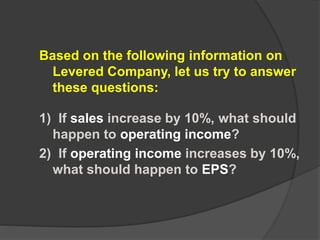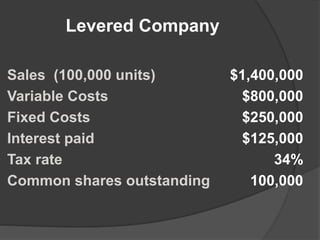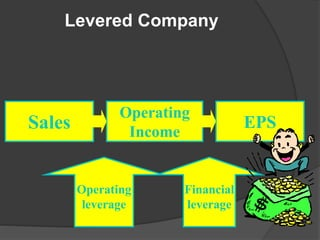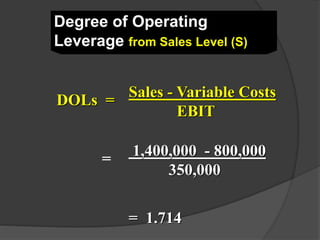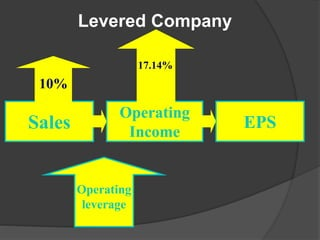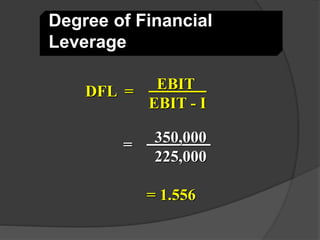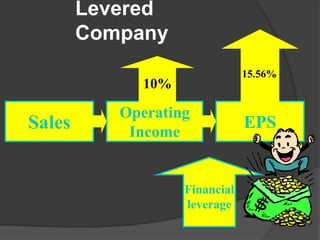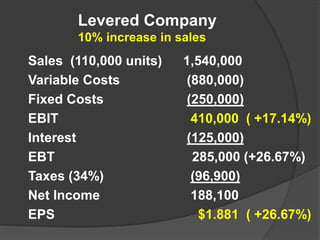1 de 24
Anúncio

### Financial leverage

1. Submitted By: Samarth Gupta 18MBAIB14 Financial Management PSMBAIBTC0204 International Centre for Cross Cultural Research & Human Resource Management
2. What is Leverage?  In general terms, leverage means the use of force and effects to produce a more than normal results from a given action  In other words, leverage is the advantage generated by using a lever  Example, using a jack to lift a car  In Finance, leverage is the use of fixed costs to magnify the potential return to a firm  2 types of fixed costs:  fixed operating costs = rent, salaries, etc.  fixed financial costs = interest costs from debt
3. What is Leverage?  Leverage can magnify returns to common stockholders but can also increase risk  Management has almost complete control over this risk introduced through the use of leverage (fixed costs)  The degree in the use of leverage depends on management’s attitude toward risk and the nature of its business, among others.  Three types of leverage with reference to the firm’s income statement:  Operating leverage,  Financial leverage, and  Combined (Total) leverage.  Leverage is measured on the profitability range of operations.
4. What is Leverage? Sales Less: Total variable Costs Contribution Margin Less: Fixed Cost Earnings Before Interest and Taxes (EBIT) Less: Interest Earnings Before Taxes Less: Taxes Earnings After Taxes (EAT) Number of Shares Outstanding Earnings Per Share Operating leverage Financial leverage
5. Operating Leverage  The use of fixed operating costs as opposed to variable operating costs.  A firm with relatively high fixed operating costs will experience more variable operating income if sales change.
6. Degree of Operating Leverage (DOL)  Operating leverage: by using fixed operating costs, a small change in sales revenue is magnified into a larger change in operating income.  This “multiplier effect” is called the degree of operating leverage.
7. DOLs = % change in EBIT % change in sales change in EBIT EBIT change in sales sales = Degree of Operating Leverage from Sales Level (S)
8.  If we have the data, we can use this formula: Degree of Operating Leverage from Sales Level (S) DOLs = Sales - Variable Costs EBIT
9. Financial Leverage  The use of fixed-cost sources of financing (debt, preferred stock) rather than variable- cost sources (common stock).
10. Financial Risk  The variability or uncertainty of a firm’s earnings per share (EPS) and the increased probability of insolvency that arises when a firm uses financial leverage. FIRMEBIT EPS Stock- holders
11. Degree of Financial Leverage (DFL)  Financial leverage: by using fixed cost financing, a small change in operating income is magnified into a larger change in earnings per share.  This “multiplier effect” is called the degree of financial leverage.
12. DFL = % change in EPS % change in EBIT change in EPS EPS change in EBIT EBIT Degree of Financial Leverage =
13. Degree of Financial Leverage  If we have the data, we can use this formula: DFL = EBIT EBIT - I
14. What does this tell us?  If DFL = 3, then a 1% increase in operating income will result in a 3% increase in earnings per share. Stock- holdersEBIT EPSSales
15. Based on the following information on Levered Company, let us try to answer these questions: 1) If sales increase by 10%, what should happen to operating income? 2) If operating income increases by 10%, what should happen to EPS?
16. Levered Company Sales (100,000 units) \$1,400,000 Variable Costs \$800,000 Fixed Costs \$250,000 Interest paid \$125,000 Tax rate 34% Common shares outstanding 100,000
17. EPS Financial leverage Operating Income Sales Operating leverage Levered Company
18. Degree of Operating Leverage from Sales Level (S) 1,400,000 - 800,000 350,000 = 1.714 = DOLs = Sales - Variable Costs EBIT
19. EPS Operating Income Sales Operating leverage 10% 17.14% Levered Company
20. Degree of Financial Leverage DFL = EBIT EBIT - I = 350,000 225,000 = 1.556
21. EPS Financial leverage Operating Income Sales 10% 15.56% Levered Company
22. Sales (110,000 units) 1,540,000 Variable Costs (880,000) Fixed Costs (250,000) EBIT 410,000 ( +17.14%) Interest (125,000) EBT 285,000 (+26.67%) Taxes (34%) (96,900) Net Income 188,100 EPS \$1.881 ( +26.67%) Levered Company 10% increase in sales
Anúncio